# 注册岩土工程师基础考试上午专家预测试卷1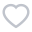63人已考
•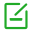卷面总分：120
•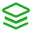试卷类型：预测试题
•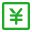测试费用： 免费
•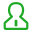关注人数：3723
•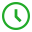作答时间：240分钟
•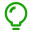解析：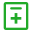试题类型：
• 1.单项选择题• 1.单项选择题
1 设a、b、c均为向量，下列命题或等式中正确的是( )。

A.若a·b=a·c，则b=c

B.若a×b=a×c，则b=c

C.a·b=b·a

D.a×b=b×a

2 一直线过点A(2，1，3)，且与直线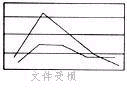垂直相交，则此直线的方程是( )。

A.B.C.D.3 下列为经过两平面x+5y+z=0，x-z+4=0的交线，且与平面x-4y-8z+12=0的夹角为x/4的平面方程的是( )。

A.x-20y+7z=12

B.x+20y+7z=12

C.x+20y-7z=12

D.x-20y-7z=12

4 空间直线在xoy坐标面上的投影曲线为( )。

A.x2+y2=1

B.x2+2y2=1

C.4-3x2-2y=0

D.5 极限的值是( )。

A.2

B.0

C.1

6 函数在x=0处下列结论中正确的是( )。

A.左导数存在，右导数不存在

B.右导数存在，左导数不存在

C.左右导数存在，但导数不存在

D.导数存在

7，则y'|x=0的值为( )。

A.-3/2

B.3/2

C.-2/3

D.-1/2

8 设曲线y=e1-x2与直线x=-1的交点为P，则曲线在点P处的切线方程是( )。

A.y-3x+1=0

B.x-y+3=0

C.2x-y-3=0

D.2x-y+3=0

9 f′(ex)=1+x，则f(x)的值为( )。

A.(1+x)lnx+c

B.lnx+c

C.D.xlnx+c

10的值为( )。

A.0

B.π

C.a

D.2a